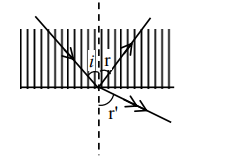# Solve this following`
Question:

A ray of light passes from a denser medium to a rarer medium at an angle of incidence $i$. The reflected and refracted rays make an angle of $90^{\circ}$ with each other. The angle of reflection and refraction are respectively $r$ and $r^{\prime}$. The critical angle is given by :1. $\sin ^{-1}(\operatorname{cotr})$

2. $\tan ^{-1}(\sin i)$

3. $\sin ^{-1}\left(\operatorname{tanr}^{\prime}\right)$

4. $\sin ^{-1}(\tan r)$

Correct Option: , 4

Solution:

$r+r^{\prime}+90^{\circ}=180^{\circ} \Rightarrow r^{\prime}=90-r=90-i$

$\mathrm{n}_{1} \sin \mathrm{i}=\mathrm{n}_{2} \sin \mathrm{r}^{\prime}=\mathrm{n}_{2} \sin (90-\mathrm{i})$

$n_{1} \sin i=n_{2} \cos i \Rightarrow \tan i=\frac{n_{2}}{n_{1}}$

Now $\sin C=\frac{n_{2}}{n_{1}}=\tan i$

$\Rightarrow \mathrm{C}=\sin ^{-1}(\tan \mathrm{i})=\sin ^{-1}(\tan \mathrm{r})$